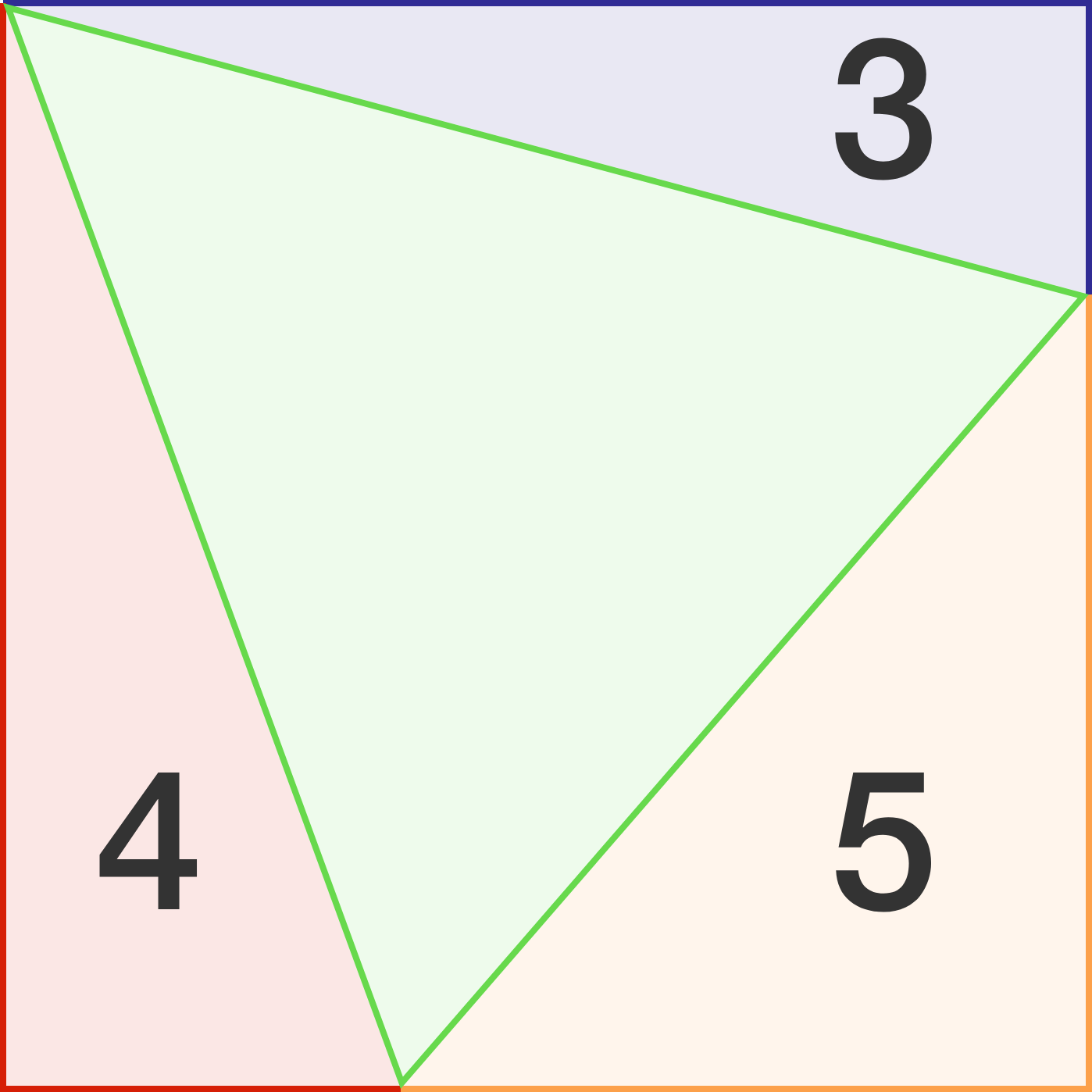# 3-4-5 Square

Geometry Level 3The diagram above illustrates a square divided into four different-colored triangles, three of them with numbers given as their respective areas.

What is the area of the whole square?

If your result can be expressed in the form $a\left(b + c\sqrt{d}\right),$ where $a,b,c,d$ are all positive integers, $\gcd(b,c) = 1,$ and $d$ is square-free, input $a + b + c + d$ as your answer.

×